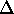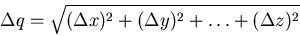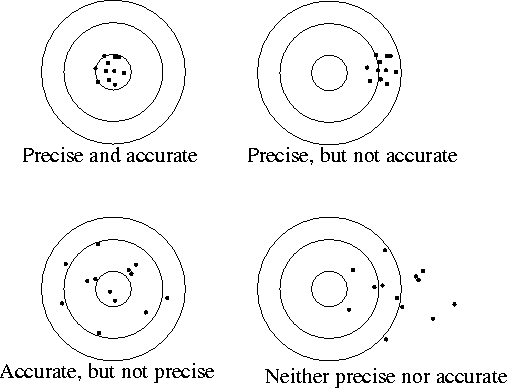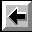# Error Analysis

Name:_______________________________ 4-digit Code Number:_______________________________

Partner(s):

Station number:

Error in this context does not mean mistake but rather refers to the uncertainty in a measurement. All measurements in practice and even in principle have some error associated with them; no measured quantity can be determined with infinite precision.

## Statistical Errors (also known as Random Errors)

Most measurements involve reading a scale. The fineness of the scale markings (how close together they are) is limited and the width of the scale lines is nonzero. In every case, the final reading must be estimated and is therefore uncertain. This kind of scale-reading error is random since we expect that half of the time the estimate will be too small, and the other half of the time the estimate will be too large. We expect that random errors should cancel on average, that is, many measurements of the same quantity should produce a more reliable estimate. Statistical errors can be controlled by performing a sufficiently large number of measurements. The error estimate on a single scale reading can be taken as half of the scale width. For example, if you were measuring length with a scale marked in millimeters, you might quote the reading as 17.0 mm ± 0.5 mm. If you measured the same length many times, you would expect the error on the measurement to decrease. This is indeed the case. The best estimate of the measured quantity is the mean or average of all the measurements. Simply add all the individual measurements together and divide by the number of measurements. The best estimate of the error associated with the mean value is called the "error on the mean" and is given by (the error on a single measurement) divided by (the square root of the number of measurements). Obviously, this will decrease as the number of measurements increases. The final reading for a quantity should be quoted as: (mean) ± (error on the mean).

## Error Propagation

If several quantities with associated random errors are given by: x ±x, y ±y, ... , z ±z, then the sum or difference is given by q ±q where q might be

q = x + y - z

and the error on q is propagated from the errors on x, y, ... , and z as followsNotice that the errors are added in quadrature, even when the quantities are being subtracted. The error always increases when adding or subtracting quantities.

### Multiplication and Division

If several quantities with associated random errors are given by: x ±x, y ±y, ... , z ±z, then the product or quotient is given by q ±q where q might be

q = x*y/z

and the error on q is propagated from the errors on x, y, ... , and z as follows## Systematic Errors

These errors are more insidious than statistical errors. Systematic errors are difficult to detect, and the sizes of systematic errors are difficult to estimate. Increasing the number of measurements has no effect on systematic errors because the error is always in the same direction (all measurements too high, or all measurements too low). Careful instrument calibration and understanding of the measurement being made are part of prevention. For example, suppose that you are using a stopwatch to time runners in the 100-meter dash. You are quite adept at making the measurement, but -- unknown to you -- the watch runs 5% fast. All times will be 5% too high. There will be no immediately obvious indication of a problem. If you happen to be familiar with the runners' normal times, you might notice that everyone seems to be having a slow day. To prevent such problems, one should calibrate the stopwatch with a known standard such as the Nation Institute of Standards and Technology's standard time service on short wave radio. The rules are: 1) the error should have one significant figure; 2) the number of decimal places in the measurement should be the same as the number of decimal places in the error. Always remember: There is no such thing as "human error". Try to find the deeper cause for any uncertainty or variation.## Data

Mark your D-cell so that you will be able to recognize it again.

Connect the black lead wire between the the COM (common) terminal of the analog volt meter and the bottom of the D-cell. Connect the red lead wire between the 3-volt maximum terminal of the analog volt meter and the top of the D-cell. Record the measured voltage with an error estimate.

## Error Analysis

Calculate the fractional error,V / V * 100% (keep one or two significant figures in the fractional error).

Connect the red lead between the 15-volt maximum terminal of the analog volt meter and the top of the D-cell. Record the measured voltage with an error estimate.

Calculate the fractional error.

Connect the red lead between the 30-volt maximum terminal of the analog volt meter and the top of the D-cell. Record the measured voltage with an error estimate.

Calculate the fractional error.

Exchange D-cells with the group across the aisle. Perform the same measurements as on the previous page. For each of the three measurements state whether or not the readings by the two groups agree, that is, do the error estimates overlap? (See Taylor page 5 if you are confused.) Make sure that you are comparing measurements for the SAME D-cell. That is, you should have your readings and their readings both for YOUR D-cell. They should have their readings and your readings both for THEIR D-cell.

 Our readings Their readings Overlap? (Y/N) 3 V max 15 V max 30 V max

List two sources of random (or statistical) error.

List two sources of systematic error.

## Conclusion

Make a general statement about the procedure for devices with multiple analog scales to obtain the lowest fractional error possible. Summarize what you have learned today (not what you have done).

## A Puzzle

Using a D-cell, a flashlight bulb, and one wire, make the bulb glow. Draw a large diagram of your method below. Make sure it is clear enough so that a five-year-old could look at your figure and light the bulb.Back to the Electricity and Magnetism Manual